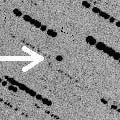# \$B>.OG@1%U%!%(%H%s(B

(3200) Phaethon (2005)###\$B###\$B50F;MWAG(B

```   The following improved orbital elements by Kenji Muraoka, are
from 116 observations 1983 to 1996, perturbations by 9 Plantes,
Moon and 5 minor planets were taken into account.
The mean residual is +/- 0.91 arc seconds.

T  =  2005 Mar.  2.53918  TT    Epoch  =  2005 Mar. 11.0  TT
Peri. =  321.98210                     e  =    0.8900199
Node  =  265.42266    (2000.0)         a  =    1.2713464 AU
Incl. =   22.17217                     n  =    0.687556270
q  =    0.1398228 AU                P  =    1.433 years
```

###\$B@1?^(B###\$B8wEYJQ2=(B

```        H = 14.3  G = 0.15
```##### \$B50F;MWAG\$OB<2,7r<#;a\$N7W;;\$K\$h\$k\$b\$N\$G\$9!#(B \$B@1?^\$O(B StellaNavigator Ver.2.0 for Windows (\$B%"%9%H%m%"!<%D(B \$BJTCx(B / \$B%"%9%-!<=PHG6I4)(B) \$B\$G:n@.\$7\$?\$b\$N\$G\$9!#(B \$B8wEY%0%i%U\$O(BComet for Windows\$B\$G:n@.\$7\$?\$b\$N\$G\$9!#(B